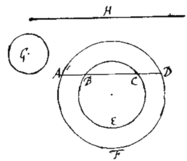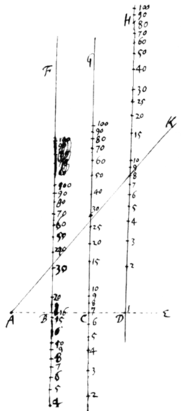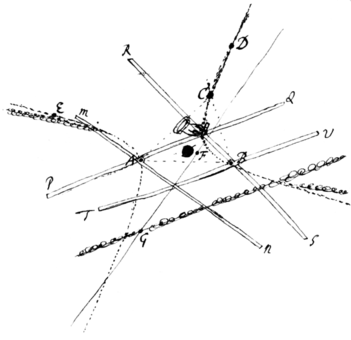<1r>

Cambridge Aug 20 1672.

Sir

Since your last I have tryed the calculation for finding by an infinite series the content of the second segments of an Ellipsoid. The first series that I met with was this $\begin{array}{ccccccccc}\begin{array}{r}\phantom{\frac{b}{0}}2rb\\ -\frac{{b}^{3}}{3r}\\ -\frac{{b}^{5}}{20{r}^{3}}\\ -\frac{{b}^{7}}{56{r}^{5}}\\ \text{&c}\end{array}\right\}& a\phantom{\frac{b}{0}}& \begin{array}{r}\phantom{\frac{0}{0}}-\frac{b}{3r}\\ -\frac{{b}^{3}}{18{r}^{3}}\\ -\frac{{b}^{5}}{40{r}^{5}}\\ \text{&c}\end{array}\right\}& {a}^{3}\phantom{\frac{0}{0}}& \begin{array}{r}\phantom{\frac{b}{0}}-\frac{b}{20{r}^{3}}\\ -\frac{{b}^{3}}{40{r}^{5}}\\ \text{&c}\end{array}\right\}& {a}^{5}\phantom{\frac{0}{0}}& \begin{array}{r}\phantom{\frac{b}{0}}-\frac{b}{56{r}^{5}}\\ \text{&c}\end{array}& {a}^{7}\phantom{\frac{0}{0}}& \begin{array}{r}\phantom{\frac{b}{0}}\text{&c.}\end{array}\end{array}$ Which upon comparison proved the same with Mr Gregories & therefore I have exprest it in the same letters. I tryed two or thre others, but could finde none more simple. Wherefore since I understand your designe is to get a rule for guageing vessells, this Problem having so bad success for that end I shall in its stead present you with this following expedient.

Let AFDA represent the vessell viewed endwiseits perimeter at the middle being AFD, & at the end BEC, & the top of the liquor ABCD. Also let G be a circle whose semidiameter is equall to the difference of the semidiameters of the other two circles AFD & BEC, & H the whole length of the vessel. Find by a table or instrument composed for that purpose, the segments AFD, & BEC, & the whole circle G; & $\frac{2}{3}AFD+\frac{1}{3}BEC-\frac{1}{15}GinH$ shall be the whole quantity of the liquor in the vessel. This rule is not exact but approaches the content of the Parabolick spindle exactly enough for practice when the op of the liquor buts upon the end of the vessell. If the vessel be just half full tis exact; if more then half full, tis something to little; if lesse than half full, too much.

The approximating the roots of affected Æquations by Gunters line is thus. Let the æquation for instance be ${x}^{3}-7xx+16x-12=0$: to resolve whichplace thre of Gunters Rulers BF, CG, & DH parallel & equally distant from one another, & to any line AE which crosseth then all apply the number 1 of the third Ruler DH, the number 7 of the second CG, & the number 16 of the first BF, accordingly as the coefficients of the æquation are. Then in the cross line taking the point A as far from B as B is from C apply any ruler to that point A, about which whilst you turne it slowly observe the numbers where it cuts the other rulers untill you see the summ of the numbers on the first & third ruler equall to the sum of the resolvend 12 & the number on the other ruler, which when it happens the number on the 3d ruler shall be the cube of the desired root. Thus in this case you see $8+32=12+28$, & therefore 8 the number on the 3d ruler is the cube of the desired root which consequently is 2.

The application of this to any æquations of higher di <1v> mensions is obvious. As also so to proportion the rulers BF, CG, DH, &c that the line AK may be carried over them with parrallel motion.

The description of a Conick section which shall pass through five given points is this. Let the five points be A, B, C, D, & E any three of which as A, B, & C joyn to make a rectilinear triangle ABC, to any two angles of which as A & B apply two sectors, their poles to the angular points, & their leggs to the sides of the triangle. And so dispose themthat they may turne freely about their poles A & B without varying the angles they are thus set at. Which done, apply to the other two points D & E successively their two leggs PQ & RS which were before applyed to C (which leggs for distinction sake may be called their describing leggs & the other two MN & TV which were applyed to AB, their directing leggs,) & marke the intersections of their directing leggs, which intersections suppose to be F when the application was made to D, & G when made to E. Draw the right line FG & produce it infinitely both ways. And then if you move the rulers in such manner that their directing leggs doe continually intersect one another at the line GF, the intersection of their other leggs shall describe the conic section which will pass through all the said five given points.

If three of the given points lye in the same streight line tis impossible for any conick section to pass through them all, And in that case you shall have instead thereof two streight lines.

Much after the same manner a Con. Sect. may be described which shall pass through 4 given points & touch a given line, or pass through 3 given points & touch two given lines, whether those lines be right or curved. &c

I presume it will not be an unpleasing speculation to your Mathematicians to find out the Demonstration of this Theorem. As also to determin the center{s}{}, diameters, axes, vertices, & Asymptotes of the Con. sect. thus described or to describe a Parabola which shall pass through 4 given points. And there I omit them.

I herewith send you a set of Problems for construing æquations which that I might not forget them I heretofore set down rudely as you'le find. And therefore I think 'em not fit to be seen by any but your selfe, which therefore you may return when you have perused them. How the afforesaid descriptions of the Conick sections are to be applyed to these constructions I need not tell you.

Borellius, of whom you desire my opinion, I esteem among the middle sort of Authors. I find not that he hath added any thing considerable to the science{s} of motion but onely proved things already evidently known. Nor hath he done that without some Paralogisms, as in the proofs of the 209th, 212th, & 260th Propositio{ns.} And some of them are not onely proved parallogistically but are also fals as <2r> the 233d & those that depend on it, but yet he may be of good use to young students in Mechanicks.

Sir I shall trouble you no further at present, but subscribe my self

I. Newton
<2v>

These to Mr John Collins

Mr Newton about the Logarithmes Rulers and Mouvable angles

From No. 4 of the selected papers.